# Implementing Regression With Gradient Descent From Scratch

This is the first post of an n-part series that involves implementing common Deep Learning architectures from scratch. The next post will be on CNNs and I’ll keep updating the links here as I progress through this project.

• Implementing Linear and Logistic Regression with Gradient Descent from Scratch (This post)
• Implementing a CNN for MNIST digit classification from Scratch (Upcoming)

I recently completed the Deep Learning Specialization from deeplearning.ai, and as usual with Coursera courses, I found myself inadequately prepared for implementing the knowledge in the real world.

While Coursera programming assignments had every student implement the same thing we’re going to do today, they spoon-feed all details to the user on a line-by-line basis, leaving very little room to think or improvise.

I’m fairly confident less than 10% of people who have completed the specialization would be able to accomplish the same assignment from scratch without taking help from online resources.

The information required to implement

• Linear Regression
• Logistic Regression

from scratch are readily available in the course, however, coding it is another ball game entirely.

The rules for this project are simple:

1. Web searches are only allowed for concepts, not for code.
2. Only low-level packages like NumPy and matplotlib are allowed.

So let’s get started!

# Logistic Regression

What’s the aim of linear regression? How does it work and why do we use it?

I’m sure these are fairly basic questions that people on the internet have explained much better than I will ever be able to.

So I’ll stick to the tricky parts. These parts have been documented as well, however, it’s best to try to understand the concepts yourself, and then implementing the code from scratch without any inspiration from anywhere.

The dataset we’ll be using is the publicly available iris dataset. Our job is to perform binary classification on the same.

The concept is simple: given a set of inputs, determine whether the output would be 0 or 1 (in case of binary classification).

How do we get the output to stick within the boundaries of 0 and 1?

The answer lies in the Sigmoid Function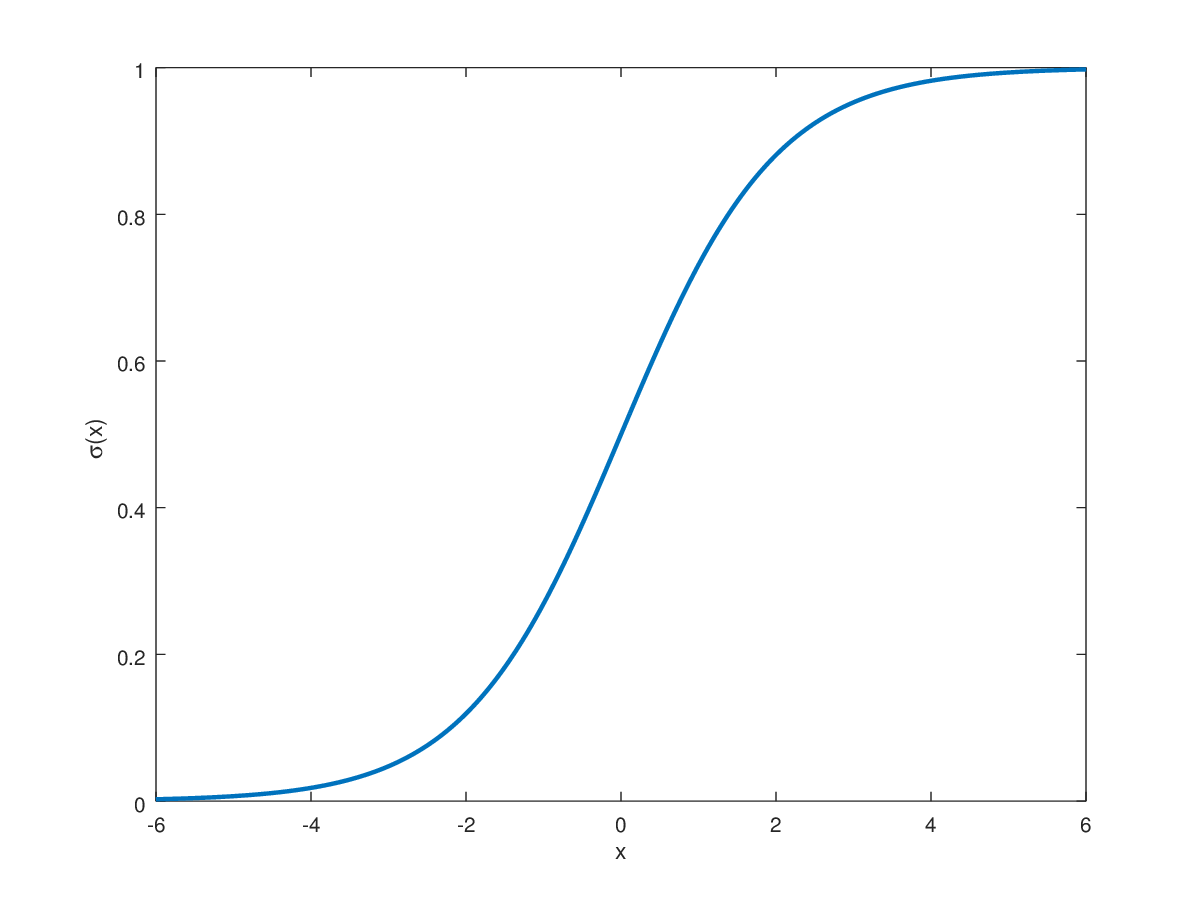Graph for the Sigmoid Curve

If you look at the graph, you’ll notice how irrespective of how large or small the input (x-axis) is, the output will always be within 0 and 1.

Talk is cheap, let’s get to the code.

`def sigmoid(x):    return np.exp(x)/(np.exp(x)+1)`

Great, so the output has been fixed. Given a set of inputs, we’ll be able to get the corresponding output.

But how do we map n number of features that would be present in the input dataset to a single value on the x-axis?

This is solved with a simple equation

Y = sigmoid(W * X + b)

Where Y represents our output, and W represents a weight vector of size (num_features,1), and X is the input vector of size (num_features, 1). The bias term is a scalar that is also trained along with the weights.

Clarification: for simplicity of the above equation, the dimensions of the actual equation have not been accounted for, but on accounting for them, the equation becomes:

Y = sigmoid (W.T * X + b)

The bias term, however, in logistic regression can often be ignored since by training the weights over a few iterations, it becomes redundant.

Great, so let’s write the function for it:

`def pred_val(X, params):    return sigmoid(np.matmul(X, params))`

Here, params represent the weight vector that was discussed earlier.

Next, to build a complete model, we’re going to need the Loss or Cost function. One approach could be to use the square root error, but that’s prone to local optima that would stop us from achieving a good rating on the test set.

The equation we’ll be using is fairly well-known, but if you want to learn why it’s so effective, this is a great resource.

L(y’,y) = - (y*log(y’) + (1-y)*log(1-y’))

Here y is the ground truth value and y’ is the predicted value.

When the ground-truth value (y) is 1, the cost function becomes:

L(y’,1) = -log(y’)

This is good because when y’ is 0 (prediction is incorrect), the cost function will be infinite (log 0 is negative ∞).

And when the ground-truth value (y) is 0, the cost function becomes:

L(y’,0) = -log(1-y’)

When y’ is 1(prediction is incorrect), the cost function ∞ again.

Let’s write the code for the same:

`def cost_fn (X, y, params):     y_pred = pred_val(X, params)        return np.sum(-(y*np.log(y_pred) + (1-y)*np.log(1-y_pred)))`

Finally, the forward propagation steps are completed. To implement the backpropagation section, we’re going to revise gradient descent for a quick sec.

• Our values for W and b should minimize the cost function.
• Our initial values for W and b will be 0 or random.
• Then we’ll keep updating them according to the learning rate as well as the derivative of W and b which is dW and db respectively (It helps to think about the derivates as the slope of the convex function. If you’re at the extremes, the slope is high and the values for the derivates will be high. Therefore, the value of W and b will change by a large value. Conversely, as you approach the convex point, the values of derivates will decrease, and W and b will not change monumentally.)

We update W and b as:

W = W - alpha * dW

b = b - alpha * db

alpha is the learning rate. The higher it is, the more the values of the gradient can affect the new values. The values can be tweaked with trial and error since higher values can lead to rapid convergence in some cases, but the opposite in other cases.

The explanation for the derivations for dW and db is something I’d better leave to those better than me, but I’m sure a quick Google search would provide you with thorough reasoning.

The equation basically boils down to:

dZ = Y_Pred - Y

dW = X * dZ

db = dZ

We’ll run this section of updating the weights n_iter number of times, which represents the number of iterations we want to run the model through.

At every iteration, we’ll calculate the cost, which we’ll plot at the end to see how well our gradient descent is working.

Finally, our last segment is complete. Let’s write the function for it:

`def gradient_descent(X, y, params, learning_rate,num_iters):        cost_history = np.zeros((num_iters, 1))    for i in range(num_iters):                y_pred = pred_val(X, params)                #dimensions of X = [num_samples, num_features]        #final dimensions have to be = [num_features, 1]        #transpose of X = [num_features, num_samples]        #dimensions of y_pred - y = [num_samples, 1]        #therefore need to premultiply the transpose of X with (y_pred - y)                derivative_of_cost = np.matmul(X.transpose(), y_pred - y)        derivative_of_cost *= learning_rate        derivative_of_cost /= len(y) #aka number of samples                params = params - derivative_of_cost                cost_history[i] = cost_fn(X, y, params)    return (cost_history, params)`

The keen-eyed would notice that we’re passing all samples to the function at once, this is done to vectorize the code and make it run exponentially faster than using for loops. We’ve tweaked our derivates and cost to divide by the number of samples to achieve the mean for the same.

Finally, the model is complete! Let’s write some basic wrapper code and look at the results: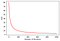Graph of the cost vs the number of iterations

We can see that in the initial iterations itself, the model begins to converge pretty fast with a value of 0.05 as alpha or the learning rate.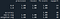Confusion matrix of results of the model

You can find the entire codebase for the implementation on my GitHub repo here.

# Linear Regression

Now that we’ve understood the logic and implementation behind logistic regression, linear regression follows along the same path.

The building blocks are the same: we have the forward and the backward pass. In the forward pass, we compute the results using matrix multiplication and during the backward pass, we’ll create a new metric for cost, since this time, it is a regression problem instead of just classification. In the simplest of terms, we don’t just have to find out if the answer is wrong, we also have to compute just how wrong the answer is.

Once we’ve written the cost function, we’ll derive new equations for the Gradient Descent, and then update our weights and biases accordingly.

This time, the dataset we’re using is from a car dealership. It takes information about the car, how many km it’s been driven, when it was released, the type of transmission, model name, etc.

When dealing with this data, however, a new problem arises.

We can only feed numerical data into our network. How do we feed textual data like model names?

Well, one solution could be to encode each model name with a separate number.

Maruti Suzuki Dzire 1
Maruti Suzuki Swift 2
Tata Nano 3

However, the problem becomes evident, since we’re going to be feeding this information in the form of matrices. The problem with numerical encoding is that it enforces a hierarchy between the data points which may not inherently exist.

Assume that the weights for our linear regression problem remain constant, and we pass two cars: Dzire and Swift.

Inherently, we have created a system where Swift would score a higher selling price than the Dzire simply because the weight matrix would be multiplied by Car_Model= 2 instead of Car_Model = 1.

This is where one-hot encoding comes into play.
Using this technique, we can create several columns that only take values of 0 or 1, where 1 implies that the object belongs to the class represented by the column.

Using One-Hot Encoding in the previous example, we get:

Maruti Dzire 1 0 0
Maruti Swift 0 1 0
(Tata) Nano 0 0 1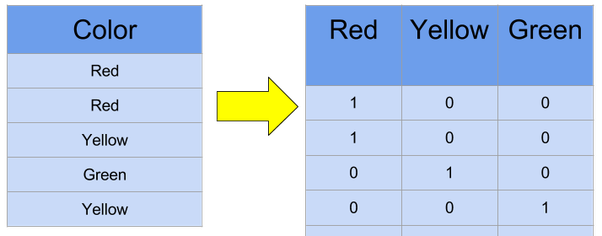Another example of the same concept (One-Hot Encoding)

With this, we create as many new columns as the number of models of the car, which can increase the size of our input data frame, but with modern CPUs and their high IPC capabilities, that shouldn’t increase computation time by any significant amount. Using one-hot encoding we have eliminated all forms of inherent ranking between categorical variables.

`df = pd.concat([df, pd.get_dummies(df['Car_Name'],prefix='Car_Name')], axis=1)df = df.drop('Car_Name', axis = 1)df = pd.concat([df, pd.get_dummies(df['Fuel_Type'],prefix='Fuel_Type')], axis=1)df = df.drop('Fuel_Type', axis = 1)df = pd.concat([df, pd.get_dummies(df['Seller_Type'],prefix='Seller_Type')], axis=1)df = df.drop('Seller_Type', axis = 1)df = pd.concat([df, pd.get_dummies(df['Transmission'],prefix='Transmission')], axis=1)df = df.drop('Transmission', axis = 1)df = pd.concat([df, pd.get_dummies(df['Owner'],prefix='Owner')], axis=1)df = df.drop('Owner', axis = 1)`

Next, we rewrite our cost function for linear regression. As mentioned before, this is different from logistic regression since we need to measure exactly how incorrect our prediction is, and the further it is from the ground truth, the more the weights and biases need to be adjusted.

For this purpose, mean squared error works perfectly. Let’s write the code for it! In this code, we’ll get the processed data from above (X), the ground truth (y) as well as the weight vector that we will be adjusting (params). First, we calculate the result by multiplying the input with the weight to get the prediction from our model, and then we check how far our prediction is from the ground truth, and return the same.

`def loss_fn(X, y, params):      #mean squared error        pred_val = np.matmul(X,params)       return np.sum((y-pred_val)**2)/len(y)   # (Summation of (y - y_pred)^2)/number of samples`

Once the cost function is ready, we move to the last complex part of this model, the gradient descent. The derivation for gradient descent is similar to that for logistic regression, simply having accounted for Mean Squared Error instead of the logistic regression loss function.

`def gradient_descent(X, y, params, learning_rate, iterations):            #grad descent means weights = weights - learning_rate * diff(COST FUNCTION)    #diff of MSE can be simplified to summation((pred_val - truth_val)*X)/number of samples    #dimensions of X: [samples*features]    #dimensions of params: [features * 1]    #dimensions of (pred_val - truth_val) [samples*1]    #to update params, final dimension has to be [features * 1]        #So, X has to be multiplied to [samples*1] and result has to be [features * 1]    # only way to do that is to premultiply X transpose [features * samples] with the above        cost_history = np.zeros((iterations,1))        for i in range(iterations):        pred_val = predict(X, params)        num_samples = len(y)        params = params - learning_rate*np.matmul(X.transpose(), pred_val - y)/num_samples        cost_history[i] = loss_fn(X, y,params)        return (cost_history, params)`

In this function, we repeat our loop for as many iterations as specified, and at each iteration, update the weights according to the MSE between the predicted and truth values.

We also log the cost of every iteration, so we can take a look at the graph of iteration vs cost, which would tell us what the ideal number of training iterations are for our model.

We also keep a holdout or test set, which is data that our model has never seen before so that during validation or testing phase, we can ensure that our model has not overfit the training data.

Let’s compile all of them together and take a look at how our code runs!Predicted vs ground truth values for our test set

As we can see from the graph above, our model seems to have adapted to the data particularly well, scoring high on even data points that it has never seen before.

Let’s take a look at the training loss over time: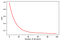Graphing the training loss vs the number of iterations

Even though we ran our model only for 100 epochs at a training rate of 0.03, it has minimized the cost very quickly. Even increasing the number of epochs would not increase computation time, since this is a very basic computation task for modern computers, but it’s still good practice to not overtrain your model.

The entire code base for linear regression can also be found on my GitHub.

With that, both of our models have been implemented. I wouldn’t call this a difficult task by any means, it’s extremely basic in the deep learning world. However, being able to do this from scratch has taught me, at the very least, about a lot of fundamentals that I used to take for granted. Manipulating data on your own, without any wrapper functions, ensures that you and only you are responsible for the success of your code.

I would encourage everyone to take this up as a challenge, it won’t take more than an hour or two.

If you’ve read so far, thank you. Feel free to leave any questions or suggestions in the comments below.

The next planned post will be about a much more challenging task: implementing CNNs using only NumPy! This will be difficult not just to implement, but also in terms of computation. I guarantee there’s a lot to learn there. Stay tuned!

## The Startup

Get smarter at building your thing. Join The Startup’s +786K followers.

Medium is an open platform where 170 million readers come to find insightful and dynamic thinking. Here, expert and undiscovered voices alike dive into the heart of any topic and bring new ideas to the surface. Learn more

Follow the writers, publications, and topics that matter to you, and you’ll see them on your homepage and in your inbox. Explore

If you have a story to tell, knowledge to share, or a perspective to offer — welcome home. It’s easy and free to post your thinking on any topic. Write on Medium